Example Questions

1 2 3 4 5 6 8 Next →

Example Question #12 : How To Divide Exponents

If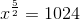, what is?Explanation:

Using the properties of exponents, we can raise both sides to a reciprocal of the exponent ofto find the value we need. Specifically...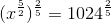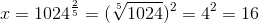Example Question #13 : How To Divide Exponents

Simplify: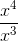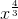Explanation:

When exponents with the same base are being divided, you may substract the exponent in the denominator from the exponent in the numerator to create a new exponent. In this case, you would subtractfrom, yieldingas the new exponent. Keeping the same base, the answer becomes.

Example Question #14 : How To Divide Exponents

What is the simplified form for the following expression?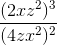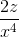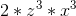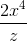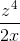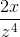Explanation:

Break up by variable.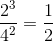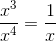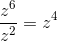Therefore the simplified form becomes,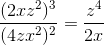.

Example Question #15 : How To Divide Exponents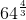can be written as which of the following?

A.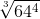B.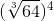C.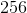C only

A and C

B only

A, B and C

B and C

A, B and C

Explanation:

C is computing the exponent, while A and B are equivalent due to properties of fractional exponents.

Remember that...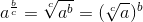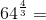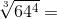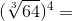Example Question #3 : How To Divide Exponents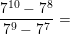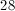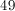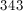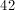Explanation:

The easiest way to solve this is to simplify the fraction as much as possible. We can do this by factoring out the greatest common factor of the numerator and the denominator. In this case, the GCF is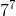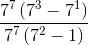Now, we can cancel out thefrom the numerator and denominator and continue simplifying the expression.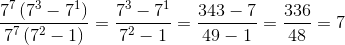1 2 3 4 5 6 8 Next →